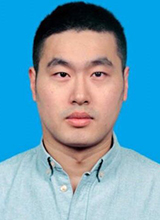# Non-uniform electric field in cantilevered piezoelectric energy harvesters: An improved distributed parameter electromechanical model

## Significance

A cantilevered piezoelectric transducer is widely used in various applications such as structural health monitoring and energy harvesting. This transducer consists of a laminated beam with elastic and piezoelectric layers and one fixed end. Based on layer arrangements, cantilevered piezoelectric transducers have three main configurations: unimorph, bimorph and multimorph, which form the basis for modeling these transducers. Based on the displacement field approximations and taking into account the transducer layer configurations, several theories have been proposed for the mathematical modeling of both cantilevered and laminated piezoelectric transducers. These studies have paved the way for extending the applications of cantilevered piezoelectric materials, specifically in energy harvesting.

Significant research effort has been made to study cantilevered piezoelectric energy harvesters. Most of these attempts, especially those based on electrochemical models, do not consider the coupling effect caused by the external circuit load, making it difficult to efficiently charge the batteries due to the complexity of the AC-to-DC conversion. To address this challenge, simple models for predicting the electrical outputs based on the resistive electrical loads have been put forwards to simplify the modeling of these piezoelectric transducers to the modeling of an electric circuit load connected to a transducer under base emotion.

Notably, traditional distributed parameter models oversimplify the electric field strength by assuming it is a uniform field. This assumption leads to two critical theoretical flows that may compromise the fundamental of piezoelectricity. First, it assumes a non-zero divergence of electric displacement of the piezoelectric layer, which contradicts the basic physical law that requires such divergence to be zero as it represents the free charge density. Second, the free charge produced by the piezoelectric layer is determined by integrating the internal electric displacements, a method that lacks theoretical support. Recent research revealed that non-uniform electric field-based models could be potentially used to predict the performance of piezoelectric structures with strong couplings. Unfortunately, the applicability of non-uniform electric fields to piezoelectric energy harvesters remains largely underexplored.

Motivated by the electric field distribution obtained by using exact solutions from plane problems, Professor Xianfeng Wang from Wuhan University of Technology and Professor Zhifei Shi from Beijing Jiaotong University proposed a non-uniform electric field. By considering both unimorph and bimorph configurations, an improved distributed parameter electromechanically model for cantilevered piezoelectric energy harvesters was proposed. The electromechanical governing equations that comprised the bending stiffness were explicitly solved to address the aforementioned theoretical issues. The work is currently published in the journal, Composite Structures.

The authors showed that the present model perfectly addressed the two theoretical flows associated with the traditional distributed parameter models. It also corrected electromagnetic characteristics of the energy harvesters produced by the traditional distribution model, including voltage output, bending stiffness, power output and resonant frequency. The bending stiffness correction was influenced by the piezoelectric layer proportion, and larger bending stiffness corrections were achieved at a higher piezoelectric ratio. At a piezoelectric ratio of about 1.0, unimorph and biomorph configurations attained a maximum bending stiffness correction of about 15.0% and 3.7%, maximum resonant frequency of 7.0% and 1.8% and maximum open-circuit voltage correction of 8.5% and 2.4%, respectively. Furthermore, a close relationship between the maximum theoretical bending stiffness correction, the piezoelectric layer parameters and the geometric dimensions of the energy harvesters was established.

In summary, an enhanced distributed parameter electrochemical model based on the non-uniform electric field assumption was proposed by Professor Xianfeng Wang and Professor Zhifei Shi for cantilevered piezoelectric energy harvesters. The non-uniform electric field was successfully validated by comparing the results of the proposed model with exact solutions of plane problems and finite element results. The corrections of the various characteristics like bending stiffness improved the overall performance of the cantilevered piezoelectric energy harvesters. In a statement to Advances in Engineering, Professor Xianfeng Wang said the study findings will advance the design of high-performance cantilevered piezoelectric energy harvesters.